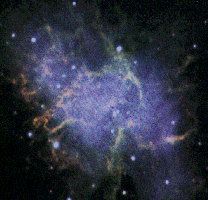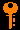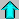# Lecture 18 - White Dwarfs & Neutron Stars (3/23/99)Supernovae --- | --- Black HolesReading:
Chapter 17-2, 18-5 (ZG4)

Notes:
pages 71-77

M1, the Crab Nebula, in the consellation Taurus. This supernova remnant harbors a pulsar. (Courtesy SEDS)
Key Question:## Investigations:

1. Neutron Stars
• What is a neutron star?
• How is a neutron star supported against gravity?
• What is the radius of a 1.4 Msun neutron star?
• What is the escape velocity of a neutron star?
• What happens to a neutron star above 3 Msun?
• Why do neutron stars often emit X-rays and gamma rays?
• How are neutron stars formed?
• How do neutron stars cool after they are formed?
• What is the interior of a neutron star like?
2. Pulsars
• What is a pulsar?
• Why do neutron stars sometimes have extreme magnetic fields?
• What do we expect for the rotation rate of a young neutron star?
• Why do pulsars "spin down" with time?
• What is the electric field on a pulsar from the rotating magnetic field?
• What is the light cylinder for a pulsar?
• What can we learn from pulsars?
3. Classical Black Holes
• Is there an object with an escape velocity equal to the speed of light?
• What is the Schwarzschild radius for a mass M?
• What is the classical gravitational redshift at the Schwarzschild radius?
• What is the gravitational tidal force at the Schwarzschild radius? How does it scale with mass?
• Why might X-rays be emitted from around a black hole?
• If the sun collapsed to a black hole, would we notice it gravitationally?

## Neutron Stars in Outline:

1. Supernova Recap
• The end result of a star's life depends upon the mass of the star:
• M < 0.01 Msun: Planet (big Jupiter)
• 0.01 < M < 0.08 Msun: Brown Dwarf (failed star)
• 0.08 < M < 0.25 Msun: Helium White Dwarf
• 0.25 < M < 8 Msun: C-N-O White Dwarf -> planetary nebula
• 8 < M < 10 Msun: O-Mg-Ne White Dwarf -> planetary nebula
• 10 < M < 40 Msun: Neutron Star -> supernova
• M > 40 Msun: Black Hole -> supernova
• SN1987A began as a 20 Msun star, evolved to a red giant of luminosity 60000 Lsun, burned increasingly heavy elements in its core, exploded in a supernova, and was observed here on Earth on February 24, 1987.
2. Neutron Stars
• Neutron stars have typical masses of 1.5 Msun within a radius of 10 km.
• Neutron stars are very hot soon after their creation, but have a tiny surface area and so are very faint.
• During collapse, conservation of angular momentum causes neutron star to spin up in rotation frequency by the inverse ratio of the radii squared.
• Also during collapse, the magnetic field is amplified by the ratio of the densities (inverse radius cubed).
• There is tremendous spin and magnetic energy in the neutron star, not to mention the gravitational energy in the immense surface gravity.
• Thus, we might hope to detect emission that taps one of these energy sources.
3. Pulsars
• In 1967, Hewish and Bell discover "pulsed" radio emission from unknown astronomical objects. These are later identified as from rapidly rotating neutron stars. Hewish later received the Nobel Prize for this discovery.
• These objects are called pulsars after the pulses received once every rotation.
• The pulses are due to a beam of radiation emitted from the magnetic poles of the pulsar (which like the Earth's are not aligned with the rotational axis) which sweep by the Earth like a searchlight.
• This radio emission comes from synchrotron radiation from high energy particles caught in the strong magnetic fields coming out of the pole of the pulsar.
• There is a pulsar in the center of the Crab Nebula. This is what provides the power for the nebula emission, through its magnetic and rotational energy.
• The Crab pulsar is rotating with a period of 0.033 seconds (!), but is slowing down due to the loss of energy to the nebula. Eventually it will be spinning too slowly to emit much radiation and will disappear from the skies.
• Currently over 500 pulsars are known, with periods ranging from just over 0.001 seconds to tens of seconds. Pulsars have been seen pulsing at optical, X-ray, and Gamma ray wavelengths. Some are even in binary systems (how did they survive the supernova?).
4. The Structure of Neutron Stars
• A neutron star is made, basically, of neutrons. It is like a giant solar-mass atomic nucleus!
• The conditions inside a neutron star are far removed from what we can study in our laboratories here on Earth. There is much interest, theorising, and speculation concerning the internal state of neutron star matter.
• However, there is some internal structure to the neutron star.
• The outer kilometer or so is a crust made of heavy nuclei (like iron) and electrons.
• Below this, there is a zone 3-5 kilometers thick of superfluid neutrons. Superfluidity is a strange state of matter where quantum effects allow bizarre behavior to occur.
• In most of the central zone of the neutron star, superfluid neutrons are joined by a small fraction of superconducting protons and electrons.
• Some astrophysicists speculate that at the heart of a neutron star is a core made of an even stranger state of matter.
• The pulsar emission taps into the magnetic and rotational energy of the neutron star. There is also a great deal of energy in the gravitational field also.
• The surface gravity of the neutron star is so great that a few grams of matter released from 1 AU away will impact the surface with the energy of a several megaton nuclear explosion!
• Gaseous material gravitationally swept up near a neutron star will be accelerated to high velocities.
• In addition tidal forces near the neutron star will be strong enough to rip apart solid or gaseous bodies, and disperse the matter into a rotating disk surrounding it.
• This accretion disk, which is similar to the protostellar disks we studied earlier, will be heated by the gravitational acceleration and tidal forces to high temperatures (millions of degrees K).
• This high temperature gas will emit X-rays, which can be seen with X-ray telescopes on Earth-orbiting satellites. We have located many neutron star systems this way.
• We have found a number of neutron-star / normal star binary systems where gas from the normal star has made its way to an accretion disk.
• Neutron stars are pretty extreme objects, but we believe there are even more extreme objects out there!

## Black Holes:

As you compress a given mass into a smaller and smaller radius, the surface gravity grows. Eventually, it will be so strong that the escape velocity will exceed the speed of light. Such an object would appear dark, since no light could escape from its surface.

The escape velocity is the velocity at which a projectile (or particle) would have to be fired straight up so that it will eventually (infinitely far in the future) escape the gravity (come to rest at zero velocity infintely far away). The escape velocity can be calculated from the energy equation:

E = mv2/2 - GMm/R

For escape, v=0 at R=infinity, so therefore in such an orbit E=0. Therefore, at the surface (or any radius R), the escape velocity is given by:

vesc2 = 2 G M / R

Note that this velocity is higher than the (circular) orbital speed given by the centripetal velocity:

vorb2 = G M / R

by a factor SQRT(2). If you increase the speed of the Earth in its orbit by more than the factor 1.414, then it would no longer be bound in orbit about the Sun and would be free to fly about the galaxy. Q: What is the escape velocity from the surface of the Earth?

Therefore, if a mass M is compressed to a radius

Rsw = GM / c2

or smaller, then the escape velocity at the radius GM/c^2 will equal the speed of light. This radius is called the Schwarzschild Radius for the astrophysicist Karl Schwarzschild who calculated it soon after the publication of Einstein's theory in 1916.

An object with a radius equal to or less than the Schwarzschild Radius GM/c^2 is called a black hole. Light, nor anything else, can ever escape the surface of such an object, and it will appear dark. Note that this calculation uses only Newton's theory for gravity. In fact, the possibility for the existence of "dark stars" was postulated as early as 1783.

The Schwarzschild radius for 1 Msun is about 3km - if the Sun were to suddenly (and inexplicably) collapse to this radius it would become a black hole - though our orbit would remain unchanged since the gravitational force depends only on the mass and distance, not the size of the mass!

The effective radius of a black hole, the Schwarzschild radius, depends only on the mass itself, not on the actual density the the mass has (beyond the fact that it must be within its own Schwarzschild radius. As you increse the mass, the radius of the black hole increases proportionally to the mass. Furthermore, since nothing can escape, even light, the mass and size of a black hole can only increase with time.

The spherical "surface" surrounding a black hole of mass M at distance of the Schwarzschild radius GM/c^2 is called the event horizon. Once within the event horizon, matter (or radiation) is lost forever from contact with the universe outside the event horizon. The event horizon is the boundary between what we can know about and what we cannot at outside the horizon. Of course, someone unlucky to be inside the event horizon of the black hole can receive news of the outside world in a one-way information transfer.

Note that degenerate objects such as white dwarfs and neutron stars shrink as mass is added, as the increased gravity compresses the matter. The radius of a 1 Msun neutron star is around 10km, while the radius of a 3 Msun neutron star is about 9km. However, the Schwarzschild radius for 1 Msun is 3 km, and thus for 3 Msun it is 9km. Thus, a 3 Msun neutron star would be within its own Schwarzschild radius and would be a black hole (and we would never know whether it had a neutron star structure). This is the Schwarzschild limit for neutron stars. Calculations show masses greater than around 3 Msun will collpase indefinitely, as neutron degeneracy cannot withstand gravity. This is similar to the Chadrasekhar limit for white dwarfs. Barring any other sort of degeneracy or quantum effect, such a mass could collapese to infinite density, but we would never know since it is within the event horizon (unless you would wish to journey there).Prev Lecture ---Next Lecture ---Astr12 Index ---Astr12 Home

smyers@nrao.edu Steven T. Myers